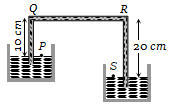A certain number of spherical drops of a liquid of radius r coalesce to form a single drop of radius R and volume V. If T is the surface tension of the liquid, then

(a)

(b)

(c)

(d)

Concept Questions :-

Surface tension
High Yielding Test Series + Question Bank - NEET 2020

Difficulty Level:

The wettability of a surface by a liquid depends primarily on

(a) viscosity

(b) surface tension

(c) density

(d) angle of contact between the surface and the liquid

Concept Questions :-

Surface tension
High Yielding Test Series + Question Bank - NEET 2020

Difficulty Level:

An engine pumps water continuously through a hose. Water leaves the hose with a velocity v and m is the mass per unit length of the water jet. What is the rate at which kinetic energy is imparted to water?

(a) $\frac{1}{2}m{v}^{3}$                                    (b) $m{v}^{3}$

(c) $\frac{1}{2}m{v}^{2}$                                    (d) $\frac{1}{2}{m}^{2}{v}^{2}$

Concept Questions :-

Bernoulli theorem
High Yielding Test Series + Question Bank - NEET 2020

Difficulty Level:

Two bodies are in equilibrium when suspended in water from the arms of a balance. The mass of one body is 36 g and its density is 9 g / cm3. If the mass of the other is 48 g, its density in g / cm3 is
(a)$\frac{4}{3}$                                         (b) $\frac{3}{2}$
(c) 3                                           (d) 5

Concept Questions :-

Archimedes principle
High Yielding Test Series + Question Bank - NEET 2020

Difficulty Level:

An inverted bell lying at the bottom of a lake 47.6 m deep has 50 cm3 of air trapped in it. The bell is brought to the surface of the lake. The volume of the trapped air will be (atmospheric pressure = 70 cm of Hg and density of Hg = 13.6 g/cm3
(a) 350 cm3                               (b) 300 cm3
(c) 250 cm3                               (d) 22 cm3

Concept Questions :-

Pressure
High Yielding Test Series + Question Bank - NEET 2020

Difficulty Level:

A siphon in use is demonstrated in the following figure. The density of the liquid flowing in siphon is 1.5 gm/cc. The pressure difference between the point P and S will be(a)                                  (b)

(c) Zero                                        (d) Infinity

Concept Questions :-

Pressure
High Yielding Test Series + Question Bank - NEET 2020

Difficulty Level:

The height of a mercury barometer is 75 cm at sea level and 50 cm at the top of a hill. Ratio of density of mercury to that of air is ${10}^{4}$. The height of the hill is
(a) 250 m                              (b) 2.5 km
(c) 1.25 km                            (d) 750 m

Concept Questions :-

Pressure
High Yielding Test Series + Question Bank - NEET 2020

Difficulty Level:

Equal masses of water and a liquid of relative density 2 are mixed together, then the mixture has a density of
(a) 2/3                                 (b) 4/3
(c) 3/2                                  (d) 3

High Yielding Test Series + Question Bank - NEET 2020

Difficulty Level:

A body of density ${\mathrm{d}}_{1}$ is counterpoised by Mg of weights of density ${\mathrm{d}}_{2}$ in air of density d. Then the true mass of the body is

(a) M                                    (b) $\mathrm{M}\left(1-\frac{\mathrm{d}}{{\mathrm{d}}_{2}}\right)$

(c) $\mathrm{M}\left(1-\frac{\mathrm{d}}{{\mathrm{d}}_{1}}\right)$                       (d) $\frac{\mathrm{M}\left(1-\mathrm{d}/{\mathrm{d}}_{2}\right)}{\left(1-\mathrm{d}/{\mathrm{d}}_{1}\right)}$

Concept Questions :-

Archimedes principle
High Yielding Test Series + Question Bank - NEET 2020

Difficulty Level:

The value of g at a place decreases by 2%. The barometric height of mercury
(a) Increases by 2%
(b) Decreases by 2%
(c) Remains unchanged
(d) Sometimes increases and sometimes decreases

Concept Questions :-

Pressure